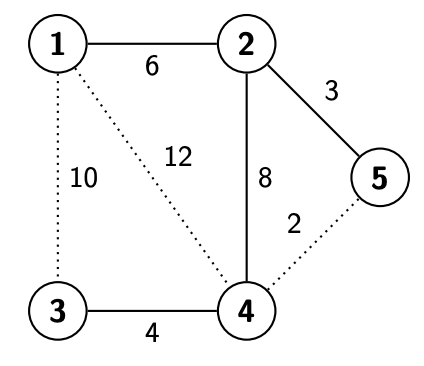시간 제한 메모리 제한 제출 정답 맞은 사람 정답 비율
3 초 1024 MB 2 2 2 100.000%

## 문제

The UltraNet company provides network connectivity for all cities in a country. For a pair of cities, they are either directly connected or indirectly connected. A city i and another city j are directly connected if a cable with a symmetrical bandwidth of bi,j = bj,i is wired between them. For two cities that are not directly connected, at least one path between the two cities exists. In the current UltraNet, more than one path is possibly available for a city pair.

The current UltraNet is perfectly working, while the maintenance fee of each cable is costly. Energy conservation is another concern. The energy consumption of a cable is proportional to its bandwidth. Therefore, the company has an optimization plan to reorganize the network with the policies in the following order:

1. The number of cables should be minimized without sacrificing the connectivity of the whole UltraNet. In other words, exactly one path between every city pair should be satisfied.
2. If there is more than one way to minimize the number of cables, the bottleneck of the whole network should be maximized. The bottleneck of a network is determined by the city pair with the narrowest bandwidth, and the bandwidth of a city pair (i, j), b'i,j, is determined by the cable with the narrowest bandwidth on the only path from i to j.
3. If there is still more than one way to meet the above two points, the total energy consumption of the network should be minimized. In other words, the sum of bandwidths of the remaining cables should be minimized.

Your task is to help UltraNet optimize the network and then output the sum of the bandwidths among all city pairs in the optimized network. For optimizing the following example, the three cables in dotted will be discarded. In the resulting network, the bottleneck is 3, the sum of bandwidths of the remaining four cables is 6 + 3 + 8 + 4 = 21, and the sum of the bandwidths among all city pairs is $$\sum_{i=1}{^n-1}\sum_{j=i+1}^{n}$$b'i,j = 6 + 4 + 6 + 3 + 4 + 8 + 3 + 4 + 3 + 3 = 44.## 입력

Each test case begins with two integers n and m, denoting numbers of cities and cables, respectively. Then, m lines will follow for specifying the m cables. Each of the m lines contains three positive integers, i, j, and bi,j, denoting that a cable with a bandwidth of bi,j directly connects the city pair (i, j), where the cities are numbered from 1 to n, and i < j since bi,j = bj,i. No more than one cable will be available between every city pair in the current network. In addition, the bandwidths of all cables are distinct; no two cables have the same bandwidth rating.

## 출력

The output is a single integer that is the sum of the bandwidths of all city pairs in the optimized network.

## 제한

• 2 ≤ n ≤ 10000
• 1 ≤ m ≤ 500000
• 1 ≤ i < j ≤ n
• 0 < bi,j < 107

## 예제 입력 1

3 3
1 2 5
1 3 6
2 3 8


## 예제 출력 1

20


## 예제 입력 2

5 7
1 2 6
1 3 10
1 4 12
2 4 8
2 5 3
3 4 4
4 5 2


## 예제 출력 2

44


## 예제 입력 3

5 5
2 5 1
1 2 2
2 3 4
1 3 5
2 4 6


## 예제 출력 3

24